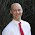# Jeromy Anglim's Blog: Psychology and Statistics

## Saturday, September 26, 2009

### Tetrachoric Correlations | Overview and Resources

What do you do if you want to run a factor analysis on a set of binary variables?
In a nutshell:
It is possible to do a standard exploratory factor analysis on binary variables. By default most programs (e.g., as in SPSS) will use the Pearson correlation as the basis for the factor analysis. Another option is to use tetrachoric correlations. The tetrachoric correlation is an option when it is assumed that there are  latent continuous variables underlying the observed binary variables. The tetrachoric correlation estimates the correlation between the assumed underlying continuous variables.

There are however a few reasons not to use tetrachoric correlations:
a) ISSUE: the resulting tetrachoric correlation matrix can be non-positive definite. This means that factor analysis will not compute (see the note here). RESPONSE: Big samples can reduce this problem. There are also ways of smoothing a correlation matrix to make it positive definite.
b) ISSUE: Garson notes that results may vary based on the method of estimation. RESPONSE: Although I have not looked into it much, any attempt to simultaneously estimate the entire correlation matrix sounds to me like a better option than the computationally simpler pair-wise approach.
c) ISSUE: Garson also notes that standard errors that assume a standard Pearson's correlation may be inaccurate. RESPONSE: If you are only concerned about getting an indication of item groupings, then standard errors tend to be less important.

Software options and to learn a little more:
John Uebersax provides the main web resource on tetrachoric correlations. He sets out a good explanation of the theory and lists ways of obtaining these correlations in various software.
Carol Woods (2002 - Factor Analysis of Scales Composed of Binary Items) also has an article on the topic.

In SPSS:
To run a factor analysis on tetrachoric correlations, you need to:
1. Calculate the tetrachoric correlations. Lorenzo-Seva and Ferrando (2012) have an article that includes SPSS macros as an online supplement for computing a tetrachoric correlation matrix.
2. Run a factor analysis using the correlation matrix from step 1. If you're not familiar with how to import a correlation matrix to use with SPSS factor analysis, check this out Z. Zhang's example.
Polychoric correlations
Much of what has been said above also relates to polychoric correlations. This is the option when the observed variables are ordinal (see Ueubersax's site for more info).

#### 3 comments:

1.If you use Ruby and SPSS, you can use statsample to create the tetrachoric correlation matrix and export it to SPSS.

http://ruby-statsample.rubyforge.org/statsample/

2.I have never done this before but do want to learn this method.

I transformed the matrix (binary items) to tetrachoric with Tetmat but I need to get the data into SPSS so I can do a principle component analysis (PCA).

Should I take the rho values in the output and write them in SPSS or how should I code the matrix?

1.Perhaps have a look at this:

Zhang, Z. (2005). Factor analysis based on correlation matrix in SPSS.

http://www.psychstat.org/us/article.php/40.htm